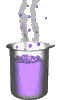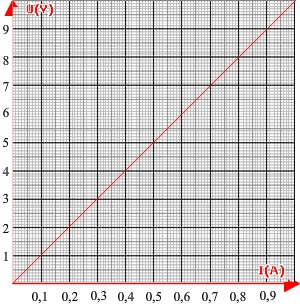Science class online
Chemistry and physics lessons

# Electricity

Free online electricity lessons for elementary school,  middle school and high school.Science class Chemistry Electricity Optics Mechanics Electricity lessons Electrical components -Two-terminal electronic components - Basic electrical components - Diodes - What's a resistor ? - How to determine a resistor value ? - Resistors effects in circuits - How to use a resistor ? - Characteristic curve of a resistor How to build simple circuits and draw diagrams - How to build a basic electrical circuit - How to draw diagrams of electric circuits The electric current - Conductors and insulators - Direction of electric current in a circuit - The dangers of electricity - Current intensity - units how to measure current ? The Voltage - The voltage and its units - How to measure a voltage ? - Voltage in open and closed circuits - Rated current and voltage for a lamp Alternating voltage and current - Alternating currents effects on led - What are alternating current and voltage ? - Periodic alternating voltage and its properties - Oscilloscope - Oscillogram - Frequency - Measuring RMS voltage with a voltmeter Serie circuits - What's a serie circuit ? - Series circuit properties - Short circuit in  series circuit - Current law in series - circuits - Voltage law in series circuits Parallel circuits - What's a parallel circuit ? - Some parallel circuits properties - Short circuits in parallel circuits - Nodes and branches in parallel circuits - Current laws in parallel circuits - Voltage laws in parallel circuits Laws of electricity - Ohm's law - Current laws in series circuits - Voltage laws in series circuits - Current laws in parallel circuits - Voltage laws in parallel circuits Generating electricity - Voltage for coil wires What is an alternator ? - Alternating currents and voltages - Generating electricity in power plants Electric power and energy - Electric power and power rating - Electric power consumption by an electrical device - Relationship between - Electric power and energy

 _____________________________________________________   _____________________________________________________

## Electrical components

### Characteristic curve of a resistor

 1) What is a characteristic curve ? Definition: A characteristic curve is the graph on which are represented the variations in the voltage at the terminals of a component as a function of the current passing through it. 2) How to draw the characteristic curve of a resistor ? To draw the characteristic curve of resistor we need the following circuit:This circuit consists of a battery providing a variable voltage, a resistor, an ammeter and a voltmeter. If the voltage of the battery changes there is a variation of voltage for the resistor and also a variation of electric current. The measuring instruments are used to determine several couples of values ​​(voltage and current). These couples are the coordinates of points used to draw the characteristic curve on a graph with the current on abscissa axis(horizontal axis) and the voltage on ordinate axis (vertical axis). ______________________________________ ______________________________________ 3) Properties of the characteristic curve of a resistor The characteristic curve of a resistor is always a line passing through the origin point. This line indicates that the voltage of the resistor is proportional to the current. The proportionality coefficient corresponds to the resistance. example: For a 10 ohm resistor we obtain, thanks to the measures, the following points: (I = 0.1 A, U = 1 V), (I = 0.2 A, U = 2 V), (I = 0.3 A, U = 3 V) (I = 0.4 A, U = 4 V) etc. These values ​​are used to draw the following graph:The voltage is ten time greater than current: ten is also the resistance. ______________________________________ ______________________________________
____________________________________

____________________________________